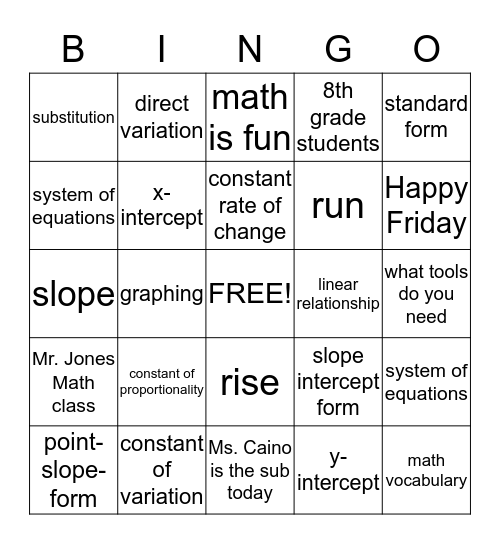# Math VocabularyThis bingo card has a free space and 24 words: constant of proportionality, constant of variation, constant rate of change, point-slope-form, standard form, substitution, system of equations, direct variation, linear relationship, rise, run, slope, slope intercept form, system of equations, x-intercept, y-intercept, what tools do you need, math is fun, Happy Friday, graphing, math vocabulary, Mr. Jones Math class, 8th grade students and Ms. Caino is the sub today.

⚠ This card has duplicate items: system of equations (2)

## Play Online